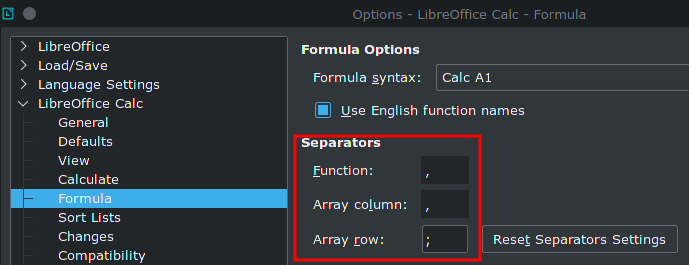# Help with bank interest calculation

I’m trying to create a spreadsheet to calculate bank interests.
There will be the following cells;
“Capital”, “Period”, “Interest”,“Tax”, “Total interest at the end of period”

I like to have the “Interest” cell dynamic, updating as according to the Capital (which is also dynamic).

So if Capital (Cell 1) is between 25.000 - 99.999 then the interest= % 13,50, if cell1 between 100.000 - 199.999 interest= %15, if cell1 between 200.000 - 499.999 then interest=17.

I want these ifs combined. So when the capital value is changed in cell1 I want the interest value to adjust accordingly.

Later I will be deducting the tax from the calculated interest value and have the proper total income.
I looked at financial calc tutorials and looked at multiple if statements but I can’t seem to achieve this.
Currently I have this broken function;
=ifa2<99999"13,50",if(A2<199000"15,00",if(A2<999999"17,00"))

try using `=LOOKUP(A2,{0;25000;100000;200000},{0;0.135;0.15;0.17})`

or `=IF(AND(A2>=25000,A2<100000),13.5%,IF(AND(A2>=100000,A2<200000),15%,IF(AND(A2>=200000,A2<500000),17%,0)))`

Hello,
Thank you so much for your respond, I really appreciate it.
Unfortunately your suggestions gives error as well.
First one gives “Err:512” and the second one gives “Err:501” and my formula gives the “Err:509”
=(

I checked the error codes from
https://help.libreoffice.org/Calc/Error_Codes_in_Calc
but I don’t know how to fix them =(

Both formulas work, if you use the default settings for an English (USA) locale:See `Tools -> Options -> LibreOffice Calc -> Formula`

And your error codes support the assumption that you have different settings here. If so, you probably need:

1. `=LOOKUP(A2;{0;25000;100000;200000};{0;0.135;0.15;0.17})` –or–
2. `=IF(AND(A2>=25000;A2<100000);13.5%;IF(AND(A2>=100000;A2<200000);15%;IF(AND(A2>=200000;A2<500000);17%;0)))`

and / or

Your decimal separator is not a `.` (dot) but a `,` (comma) and you need to change
`0.15``0,15` and
`0.17``0,17`

Great catch, thank you!
My settings are as follows;

Function ;
Array Column .
Array row ;

I’m afraid to change these to default because I have many other functioning files with these settings.

So I tried to changed the formula to;

=IF(AND(A2>=25000A2<99999);13.5;IF(AND(A2>=100000A2<199999)0;15;IF(AND(A2>=200000A2<499999);17;0)))

But I’m still getting Err:509
Would you be so kind to tailor the formula according to my settings?

UPDATE2: I’m really lost over here, no matter which comma I change I’m getting err:509 =(

Watch very, very carefully!!! You lost separators during copy&paste
e.g. `...A2>=25000A2...` should be `...A2>=25000;A2...`

(happens several times)

And no - I won’t tailor anything. Try to understand what is does and adapt to your settings.

1 Like

OH! I swear there were no commas there in the original post, I edited that one. The user must have updated their reply. Should have used yours.

Anyways, after adjusting a few more places the function now seems to work. Thank you so much.

shorter? and with ‘error handling’ - `=IF(A2>499999;“too much”;IF(A2>199999;17%;IF(A2>99999;15%;IF(A2>=25000;13,5%;“debt too small”))))’
and … if you don’t want to become crazy … avoid fractions (1234,12 \$), calculate with 123412 cent instead and divide by 100 for final results …

Thank you so much for your input.
it is not a debt or loan interest, it’s a term deposit yield thingy…

I somehow managed to make the function work for this cell… but my overall calculations do not match the bank’s amounts… I know there are dedicated financial functions but I can’t seem to find one that works for me.

What I essentially need is a 32 days term yield/interest calculation which dynamic interest rates and dynamic tax rate.

My current sheet gives me approximate amounts for now…
But thank you

@merket Sorry I’m a bit late to the party but you may find the difference between your calculation and the bank’s is the manner in which some institutions calculate interest. there are major variations of 360 days a year and 365 days a year - 360 makes an assumption of the average month being 30 days whereas we all know there are 365 days in 3 years out of four. This may help https://www.adventuresincre.com/lenders-calcs/